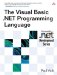# Decimal Data Type

 <  Day Day Up  >

The data type Decimal differs from the integer data types in that it can represent fractional values; it differs from the floating-point data types in that it is an exact representation of its value. Thus, Decimal is most useful in situations where fractional values are needed but exact precision is important, such as representing monetary values. Like floating-point numbers , Decimal has a whole part, a mantissa, and an exponent (also called its scale ). The default value of the Decimal type is the literal 0.0D .

While Decimal has advantages in representation over floating-point types, it also has several drawbacks. It is significantly bigger than the floating-point types: While a Double variable takes up 8 bytes, a Decimal variable takes up 16 bytes ”twice as much space. Also, most platforms do not have dedicated hardware support for Decimal operations, unlike the floating-point types, which are usually handled by a special floating-point processor. As a result, Decimal operations will be slower in most cases than floating-point operations.

## Compatibility

Previous versions of Visual Basic supported both a Decimal and a Currency data type. The Currency data type also had a fixed precision but was only eight bytes. Because it had a smaller range than Decimal , it was dropped in Visual Basic .NET in favor of Decimal .

#### Decimal Literals

Decimal literals can be specified in the same way as floating-point literals, with the exception that they must be followed by the type character D.

## Style

The character @ can be used as a type character for Decimal (in previous versions it was used for the Currency type), but its inclusion is for historical reasons, and its use is discouraged.

The following shows some examples of Decimal literals.

` Dim a, b, c, d As Decimal a = 1.5D b = 3E-30D c = 4D d = 0.4D `
 <  Day Day Up  >The Visual Basic .NET Programming Language
ISBN: 0321169514
EAN: 2147483647
Year: 2004
Pages: 173
Authors: Paul Vick

Similar book on Amazon